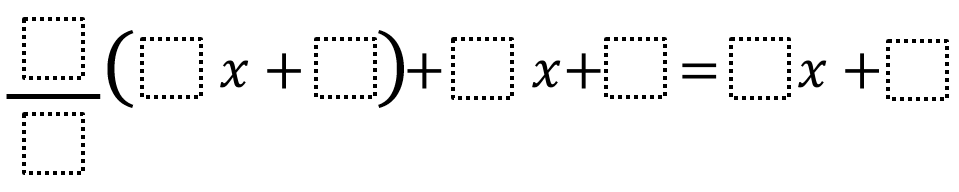Home > Grade 6 > Expressions & Equations > Equivalent Expressions 3

# Equivalent Expressions 3

Directions:  Using the whole numbers 1-9, fill in the boxes to create two equivalent expressions. You may use each whole number at most once.### Hint

Which part of the expression does the fraction affect?

There are many possibilities, here are some possibilities:

(1/2)(6x + 8) + 4x + 5 = 7x + 9
(1/2)(8x + 6) + 5x + 4 = 9x + 7

Source:  Will Case

## Subtracting Multi-Decimals

Directions: Use the digits 1 to 9, at most one time each, to fill in …